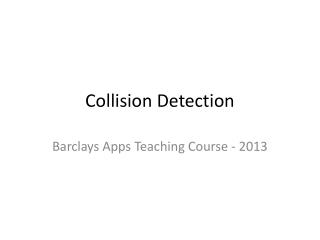DownloadDownload PresentationCollision Detection

# Collision Detection

Download Presentation## Collision Detection

- - - - - - - - - - - - - - - - - - - - - - - - - - - E N D - - - - - - - - - - - - - - - - - - - - - - - - - - -
##### Presentation Transcript

1. Collision Detection Barclays Apps Teaching Course - 2013

2. Collisions • Collision detection typically refers to the computational problem of detecting the intersection of – from two or more objects • Interactive games use collision detection • Can be done by Objects or Using Images

3. Collision Detection • Point and Rectangle • If you want to know if a point is in a rectangle – say a button w (x,y) h (px,py)

4. Point in Rectangle • You know the X and Y of the point = px and py • You know the Location X and Y of the Rectangle and also the Width and Height = x,y ,w,h • Inside =((px>=x)And(px<=(x+w-1))And(py>=y)And(py<=(y+h-1)))

5. Images • Images are a series of numbers in a file • Each number is a pixel value – colour 1100, 1222,22112,22112,3443,222322,444,3223,21222,4332

6. Images Detect if point is in Image • Similar approach – but search through the image and detect what the colour is w (x,y) (px,py)